# Environments

Tips for navigating the slides:
• Press O or Escape for overview mode.
• Visit this link for a nice printable version
• Press the copy icon on the upper right of code blocks to copy the code

### Class outline:

• Multiple environments
• Environments for HOFs
• Local names
• Function composition
• Self-referencing functions
• Currying

## Multiple Environments

### Life cycle of a function

What happens?
Def statement
``````
def square( x):
return x * x
``````
• A new function is created!
• Name bound to that function in the current frame.
Call expression
``````
square( 2 + 2)
``````
• Operator & operands evaluated
• Function (value of operator) called on arguments (values of operands)
Calling/applying
4
def square( x )
16
• A new frame is created!
• Parameters bound to arguments
• Body is executed in that new environment

### A nested call expression

1. next prev prev prev prev prev prev prev next prev next next prev
2. next next
3. next next next next next next next prev prev prev
``````
def square(x):
return x * x
square(square(3))
``````
Global frame
 square ⚫️ ----> func square(x) [parent=Global]
f1: square [parent=Global]
 x 3 Return value 9
f2: square [parent=Global]
 x 9 Return value 81

### Multiple environments in one diagram!

``````
def square(x):
return x * x
square(square(3))
``````
Global frame
 square ⚫️ ----> func square(x) [parent=Global]
f1: square [parent=Global]
 x 3 Return value 9
f2: square [parent=Global]
 x 9 Return value 81
1
2
1
2
1

An environment is a sequence of frames.

• Environment: Global frame
• Environment: Local frame (f1), then global frame
• Environment: Local frame (f2), then global frame

### Names have no meanings without environments

``````
def square(x):
return x * x
square(square(3))
``````
Global frame
 square ⚫️ ----> func square(x) [parent=Global]
f1: square [parent=Global]
 x 3 Return value 9
f2: square [parent=Global]
 x 9 Return value 81
1
2
1
2
1

Every expression is evaluated in the context of an environment.

A name evaluates to the value bound to that name in the earliest frame of the current environment in which that name is found.

### Names have different meanings in different environments

``````
def square(square):
return square * square
square(4)
``````
Global frame
 square ⚫️ ----> func square(square) [parent=Global]
f1: square [parent=Global]
 square 4 Return value 16
1
2
1

Every expression is evaluated in the context of an environment.

A name evaluates to the value bound to that name in the earliest frame of the current environment in which that name is found.

## Environments for higher-order functions

### Review: Higher-order functions

#### A higher-order function is either...

• A function that takes a function as an argument value
`summation(5, lambda x: x**2)`
• A function that returns a function as a return value
`make_adder(3)(1)`

Functions are first class: Functions are values in Python.

### Example: Apply twice

``````
def apply_twice(f, x):
return f(f(x))

def square(x):
return x ** 2

apply_twice(square, 3)
``````

### Arguments bound to functions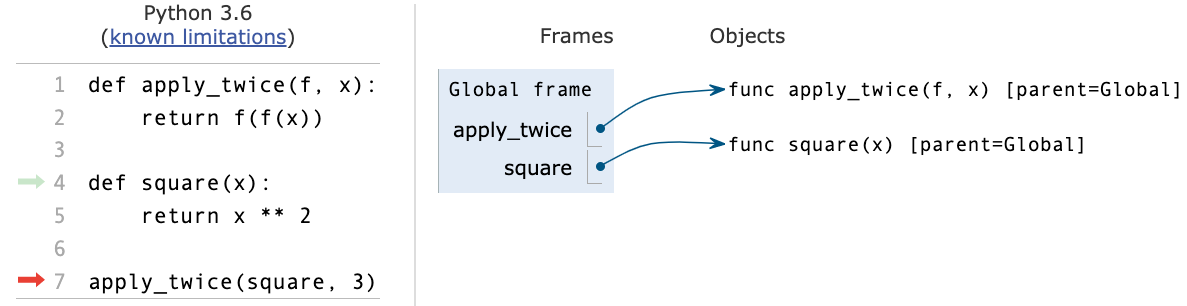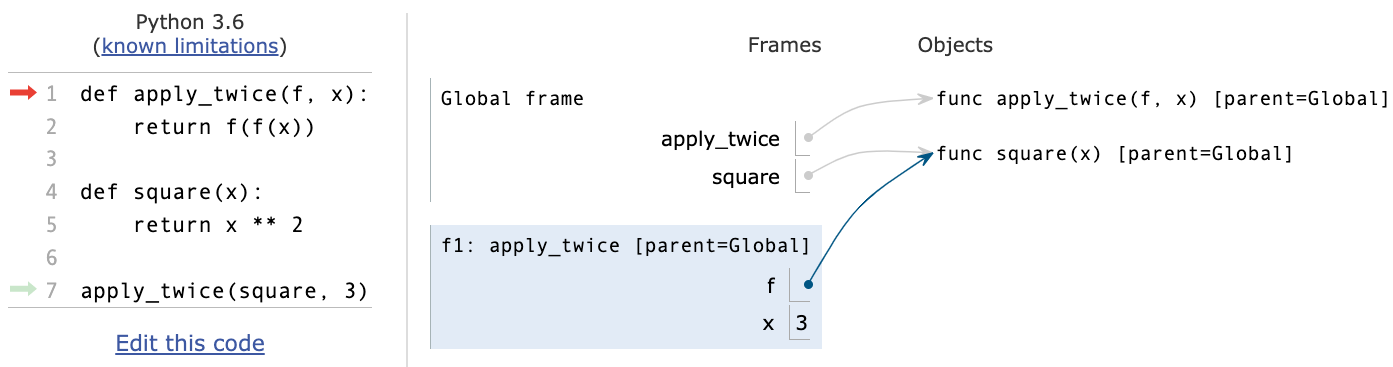2
1

## Environments for nested definitions

### Example: Make texter

``````
def make_texter(emoji):
def texter(text):
return emoji + text + emoji
return texter

happy_text = make_texter("😄")
result = happy_text("lets go to the beach!")
``````

### Environments for nested def statements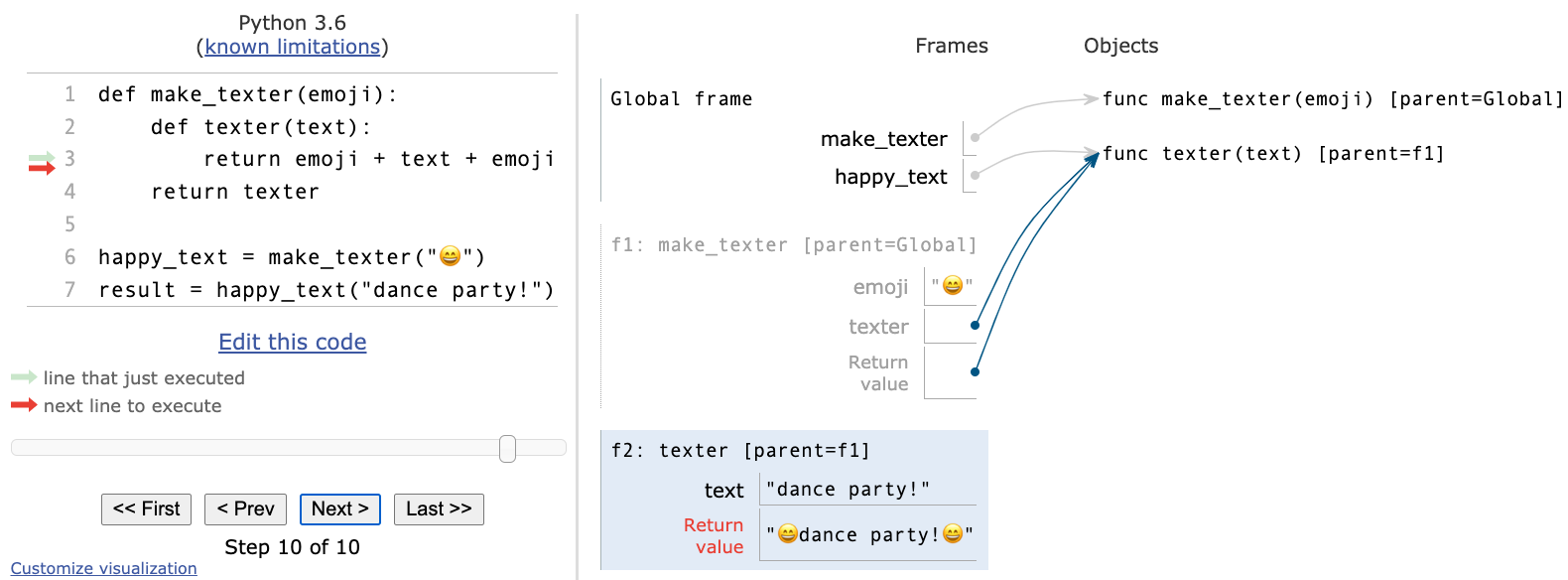3
2
1
• Every user-defined function has a parent frame
• The parent of a function is the frame in which it was defined
• Every local frame has a parent frame
• The parent of a frame is the parent of the called function
• An environment is a sequence of frames.

### How to draw an environment diagram

#### When a function is defined:

1. Create a function value:
`func <name>(<formal parameters>) [parent=<label>]`
2. Its parent is the current frame.
3. Bind `<name>` to the function value in the current frame

#### When a function is called:

1. Add a local frame, titled with the `<name>` of the function being called.
2. Copy the parent of the function to the local frame: `[parent=>label<]`
3. Bind the `<formal parameters>` to the arguments in the local frame.
4. Execute the body of the function in the environment that starts with the local frame.

## Local names

### Example: Thingy Bobber

``````
def thingy(x, y):
return bobber(y)

def bobber(a):
return a + y

result = thingy("ma", "jig")
``````

🤔 What do you think will happen?

### Local name visibility

Local names are not visible to other (non-nested) functions.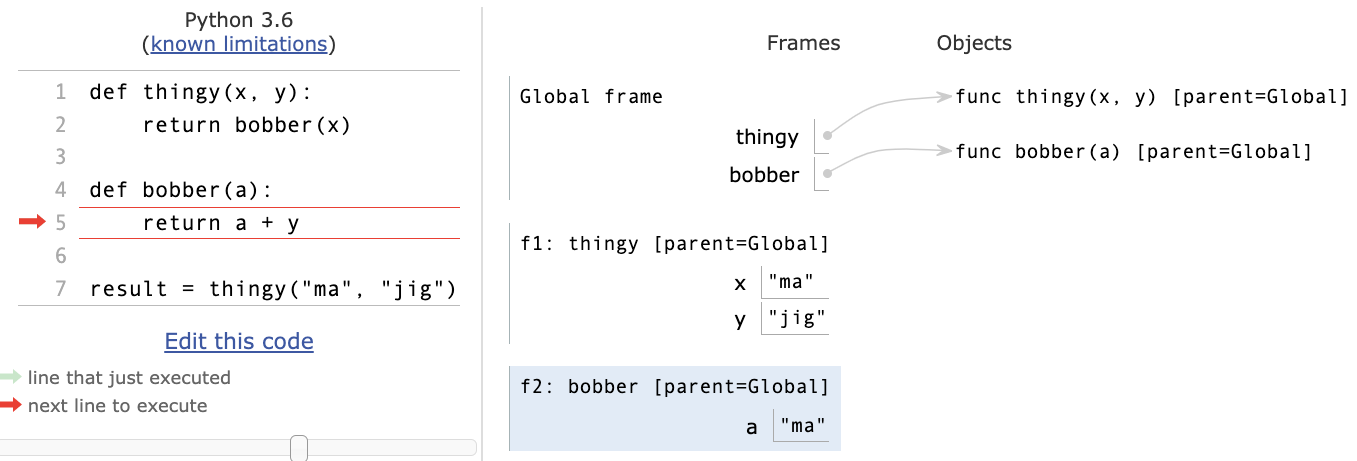• An environment is a sequence of frames.
• The environment created by calling a top-level function consists of one local frame followed by the global frame.

## Function Composition

### Example: Composer

``````
def happy(text):
return "☻" + text + "☻"

return "☹" + text + "☹"

def composer(f, g):
def composed(x):
return f(g(x))
return composed

``````

🤔 What do you think will happen?

### Example: Composer (Part 2)

One of the composed functions could itself be an HOF...

``````
def happy(text):
return "☻" + text + "☻"

return "☹" + text + "☹"

def make_texter(emoji):
def texter(text):
return emoji + text + emoji
return texter

def composer(f, g):
def composed(x):
return f(g(x))
return composed

composer(happy, make_texter("☃︎"))('snow day!')
``````

## Self-reference

### A self-referencing function

A higher-order function could return a function that references its own name.

``````
def print_sums(n):
print(n)
def next_sum(k):
return print_sums(n + k)
return next_sum

print_sums(1)(3)(5)
``````

### Environment for print_sums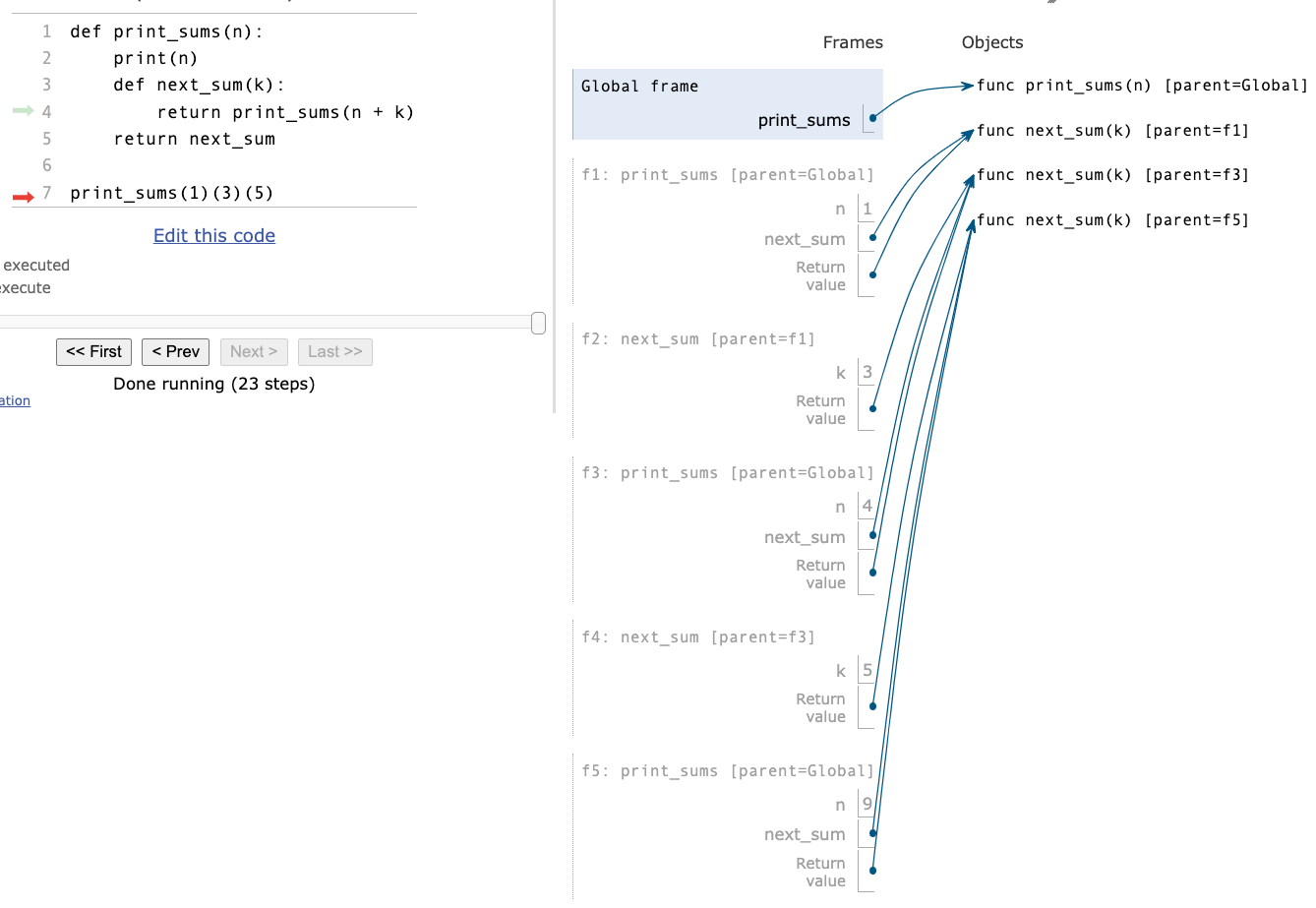### Understanding print_sums

The call:

``````
print sums(1)(3)(5)
``````

produces the same result as:

``````
g1 = print sums(1)
g2 = g1(3)
g2(5)
``````

A call to `print sums(x)` returns a function that:

• Prints `x` as a side-effect, and
• Returns a function that, when called with argument `y`, will do the same thing, but with `x+y` instead of `x`.

So these calls will...

• First print 1 and return `g1`,
• which when called with 3, will print 4 (= 1+3) and return `g2`,
• which when called with 5, will print 9 (= 4+5), and return. . . .

## Currying

Compare...

``````

``````

``````
return lambda x: n + x

``````

🤔 What's the relationship between `add(2, 3)` and `make_adder(2)(3)`?

### Function currying

Currying: Converting a function that takes multiple arguments into a single-argument higher-order function.

A function that currys any two-argument function:

``````
def curry2(f):
def g(x):
def h(y):
return f(x, y)
return h
return g
``````
``````
``````